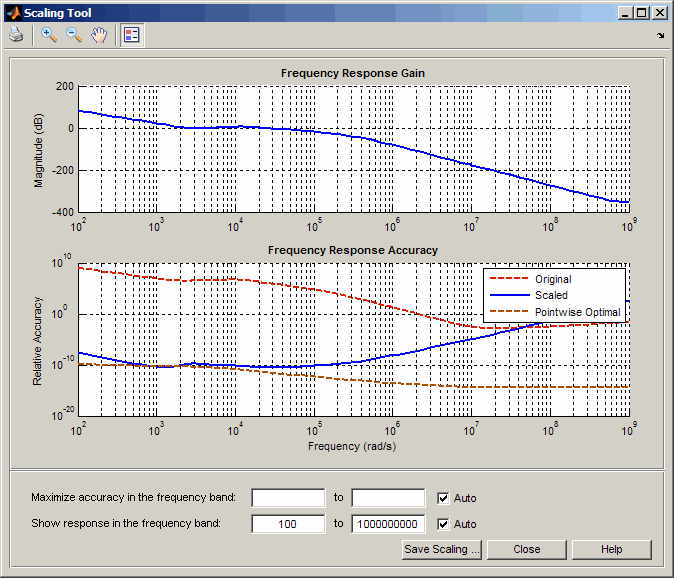Documentation

# prescale

Optimal scaling of state-space models

## Syntax

```scaledsys = prescale(sys) scaledsys = prescale(sys,focus) [scaledsys,info] = prescale(...) prescale(sys) ```

## Description

`scaledsys = prescale(sys)` scales the entries of the state vector of a state-space model `sys` to maximize the accuracy of subsequent frequency-domain analysis. The scaled model `scaledsys` is equivalent to `sys`.

`scaledsys = prescale(sys,focus)` specifies a frequency interval `focus` = {`fmin`,`fmax`} (in `rad/TimeUnit`, where `TimeUnit` is the system's time units specified in the `TimeUnit` property of `sys`) over which to maximize accuracy. This is useful when `sys` has a combination of slow and fast dynamics and scaling cannot achieve high accuracy over the entire dynamic range. By default, `prescale` attempts to maximize accuracy in the frequency band with dominant dynamics.

`[scaledsys,info] = prescale(...)` also returns a structure `info` with the fields shown in the following table.

 `SL` Left scaling factors `SR` Right scaling factors `Freqs` Frequencies used to test accuracy `RelAcc` Guaranteed relative accuracy at these frequencies

The test frequencies lie in the frequency interval `focus` when specified. The scaled state-space matrices are

`$\begin{array}{l}{A}_{s}={T}_{L}A{T}_{R}\\ {B}_{s}={T}_{L}B\\ {C}_{s}=C{T}_{R}\\ {E}_{s}={T}_{L}E{T}_{R}\end{array}$`

where TL = diag(SL) and TR = diag(SR). TL and TR are inverse of each other for explicit models (E = [ ]).

`prescale(sys)` opens an interactive GUI for:

• Visualizing accuracy trade-offs for `sys`.

• Adjusting the frequency interval where the accuracy of `sys` is maximized.For more information on scaling and using the Scaling Tool GUI, see Scaling State-Space Models.

## Tips

Most frequency-domain analysis commands perform automatic scaling equivalent to `scaledsys = prescale(sys)`.

You do not need to scale for time-domain simulations and doing so may invalidate the initial condition `x0` used in `initial` and `lsim` simulations.